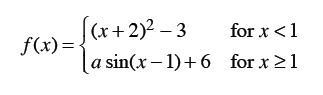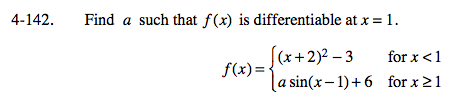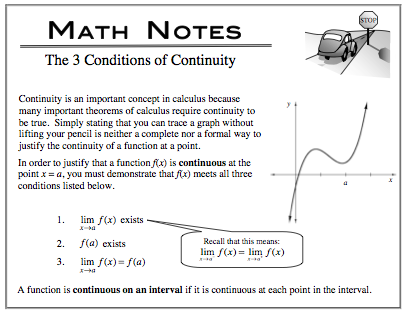### Home > CALC > Chapter 4 > Lesson 4.4.2 > Problem4-142

4-142.
1. Find a such that f(x) is differentiable at x = 1. Homework Help ✎In order to prove that f(x) is differentiable at x = 1, you must demonstrate that the derivatives agree from the left and right.

But do not forget that 'differentiablity implies continuity'. In other words, if the function is not continuous at x = 1, then it CANNOT be differentiable (even if the derivatives agree). So use the Three Conditions of Continuity to demonstrate that f(x) is continuous at x = 1.Use the eTool below to visualize the problem.
Click the link at right for the full version of the eTool: Calc 4-142 HW eTool